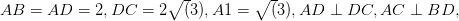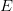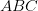# 定量生活在新加坡

But knowledge is a tricky thing to have in Asia. 如果你知道你的专业知识, 它可以在时间上你适得其反. 除非你小心, 其他人会照顾你的专业知识优势，倾倒在你自己的责任. 你可能不介意，只要他们尊重你的专业知识. 但, 他们往往占着信用为您的工作和介绍他们的逃避工作人员管理技能的能力. 人们经理 (谁可能实际上并不知道多少) 不得到更好的补偿. 这个悖论是定量生活在新加坡的一个事实. 即金融工程师享受并不一定转化为这里暴富的羡慕.国考被中国教育部门的预录入学生设置如图所示, 方形棱镜$ABCD-A_1B_1C_1D_1,$$AB=AD=2, DC=2\sqrt(3), A1=\sqrt(3), AD\perp DC, AC\perp BD,$ 和垂直的脚$E$, 证明:$BD\perp A_1C$ 确定这两个平面之间的角度$A_1BD$ 和$BC_1D$ 确定由线条所形成的角度$AD$ 和$BC_1$ 它们是在不同的平面.诊断测试通过的一年级学生在图中英文学校确定 (不按比例绘制), 角$ABC$ 为直角,$AB = 3m$$BC = 4m$ 什么是长度$AC$? 什么是三角形的面积$ABC$ (以上)? 是什么角度的黄褐色$ABC$ (以上) 以分数?

Photo by hslo